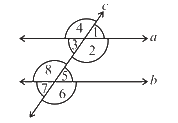# Ex.5.2 Q2 Lines and Angles - NCERT Maths Class 7

Go back to  'Ex.5.2'

## Question

(i) The pairs of corresponding angles.

(ii) The pairs of alternate interior angles.

(iii) Pairs of interior angles on the same side of the transversal.

(iv) The vertically opposite angles.Video Solution
Lines & Angles
Ex 5.2 | Question 2

## Text Solution

Steps:

(i) $$\angle1$$ and $$\angle5$$; and $$\angle2$$ and $$\angle6$$;$$\angle4$$ and $$\angle8$$;$$\angle3$$ and $$\angle7$$;

(ii) $$\angle3\rm \,and\,\angle5 \,, \angle2 \,and\,\angle8$$

(iii) $$\angle3\,{\text {and} }\,\angle8,\angle2\,{\text {and} }\,\angle5$$

(iv) $$\angle1$$ and $$\angle3$$$$\angle2$$ and $$\angle4$$$$\angle6$$ and $$\angle8$$$$\angle5$$ and $$\angle7$$

Learn from the best math teachers and top your exams

• Live one on one classroom and doubt clearing
• Practice worksheets in and after class for conceptual clarity
• Personalized curriculum to keep up with school